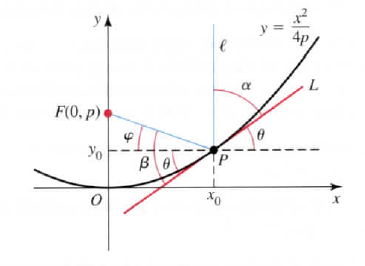×
Get Full Access to Calculus: Early Transcendentals - 1 Edition - Chapter 10.4 - Problem 83e
Get Full Access to Calculus: Early Transcendentals - 1 Edition - Chapter 10.4 - Problem 83e

×

# (See the Guided Projects for additional applications ofISBN: 9780321570567 2

## Solution for problem 83E Chapter 10.4

Calculus: Early Transcendentals | 1st Edition

• Textbook Solutions
• 2901 Step-by-step solutions solved by professors and subject experts
• Get 24/7 help from StudySoup virtual teaching assistantsCalculus: Early Transcendentals | 1st Edition

4 5 1 334 Reviews
10
5
Problem 83E

(See the Guided Projects for additional applications of conic sections.)

Reflection property of parabolas Consider the parabola $$y=x^{2} /(4 p)$$ with its focus at F(0, p) (see figure). We know that light is reflected from a surface in such a way that the angle of incidence equals the angle of reflection. The goal is to show that the angle between the ray $$\ell$$ and the tangent line L ($$\alpha$$ in the figure) equals the angle between the line PF and L ($$\beta$$ in the figure). If these two angles are equal, then the reflection property is proved because $$\ell$$ is reflected through F.

a. Let $$P\left(x_{0}, y_{0}\right)$$ be a point on the parabola. Show that the slope of the line tangent to the curve at P is $$\tan \theta=x_{0} /(2 p)$$.

b. Show that $$\tan \varphi=\left(p-y_{0}\right) / x_{0}$$.

c. Show that $$\alpha=\pi / 2-\theta$$; therefore, $$\tan \alpha=\cot \theta$$.

d. Note that $$\beta=\theta+\varphi$$. Use the tangent addition formula

$$\tan (\theta+\varphi)=\frac{\tan \theta+\tan \varphi}{1-\tan \theta \tan \varphi}$$ to show that $$\tan \alpha=\tan \beta=2 p / x_{0}$$.

e. Conclude that because $$\alpha$$ and $$\beta$$ are acute angles, $$\alpha=\beta$$.Step-by-Step Solution:

Solution 83EStep 1 of 5:a) In this problem we need to Show that the slope of the line tangent to the curve at P is tan(.Given parabola is y = with its focus at F (0 , p).Let p() be a point on the parabola.Let be an inclination of the tangent line , then the slope of the tangent line is tan(We know that ,slope is denoted by = m = tan(Consider , y = then differentiate both sides with respect to x we get , = () = , since cwhere c is constant. = .Therefore , the slope of the tangent line at the point p() is : = m = tan(=

Step 2 of 5

Step 3 of 5

##### ISBN: 9780321570567

The full step-by-step solution to problem: 83E from chapter: 10.4 was answered by , our top Calculus solution expert on 03/03/17, 03:45PM. Calculus: Early Transcendentals was written by and is associated to the ISBN: 9780321570567. The answer to “?(See the Guided Projects for additional applications of conic sections.)Reflection property of parabolas Consider the parabola $$y=x^{2} /(4 p)$$ with its focus at F(0, p) (see figure). We know that light is reflected from a surface in such a way that the angle of incidence equals the angle of reflection. The goal is to show that the angle between the ray $$\ell$$ and the tangent line L ($$\alpha$$ in the figure) equals the angle between the line PF and L ($$\beta$$ in the figure). If these two angles are equal, then the reflection property is proved because $$\ell$$ is reflected through F.a. Let $$P\left(x_{0}, y_{0}\right)$$ be a point on the parabola. Show that the slope of the line tangent to the curve at P is $$\tan \theta=x_{0} /(2 p)$$.b. Show that $$\tan \varphi=\left(p-y_{0}\right) / x_{0}$$.c. Show that $$\alpha=\pi / 2-\theta$$; therefore, $$\tan \alpha=\cot \theta$$.d. Note that $$\beta=\theta+\varphi$$. Use the tangent addition formula$$\tan (\theta+\varphi)=\frac{\tan \theta+\tan \varphi}{1-\tan \theta \tan \varphi}$$ to show that $$\tan \alpha=\tan \beta=2 p / x_{0}$$.e. Conclude that because $$\alpha$$ and $$\beta$$ are acute angles, $$\alpha=\beta$$.” is broken down into a number of easy to follow steps, and 177 words. Since the solution to 83E from 10.4 chapter was answered, more than 354 students have viewed the full step-by-step answer. This textbook survival guide was created for the textbook: Calculus: Early Transcendentals, edition: 1. This full solution covers the following key subjects: show, tan, angle, Tangent, figure. This expansive textbook survival guide covers 112 chapters, and 5248 solutions.

Unlock Textbook Solution